# Using Basic Functions in Microsoft Excel

This tutorial introduces Excel functions, which are a little like templates for common formulas. There are many different types of functions. First, we will look at the SUM function. You will learn about using AutoComplete for entering formulas. We'll look at other basic common functions such as AVERAGE. We'll take a look at the Formulas tab introduced in the Ribbon.

For more on MS Excel training classes in Los Angeles call us on 888.815.0604.

#### Formulas vs. Functions

To open the Insert Function dialog box, use the following procedure.

1. Select the Insert Function tool right next to the Formula Bar.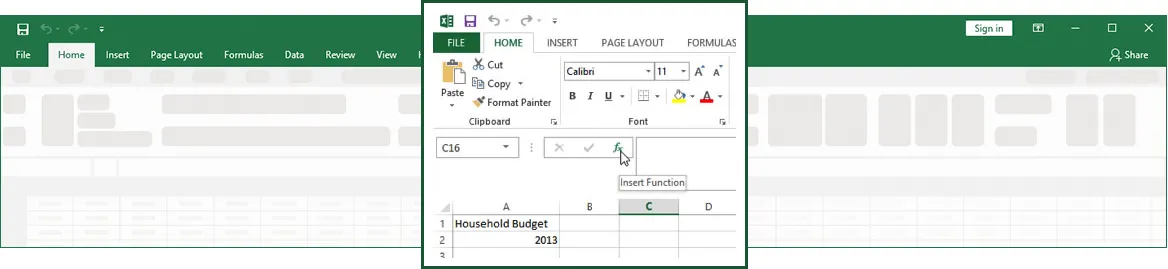Investigate the different categories and functions in the Insert Function dialog box. Point out the bottom part of the screen where the syntax and description of the function appear.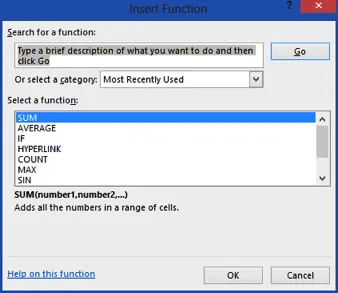#### Using the SUM Function

Review how to use a SUM function to add the total for each category in the sample file, use the following procedure.

1. Select the Total –First Six Months column for the first category (cell H5).
2. Select the AutoSum tool in the Editing Group on the Home tab of the Ribbon.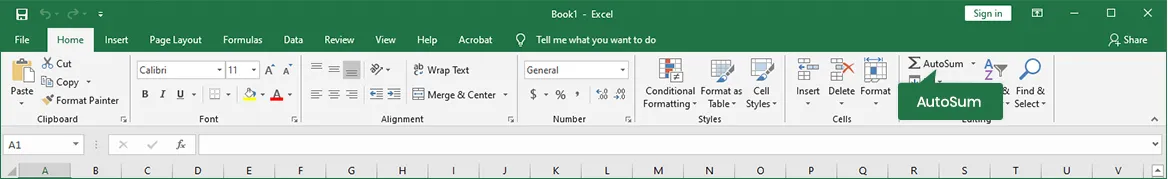1. Excel enters the function with a default selection of the cell references you want to use in the function highlighted.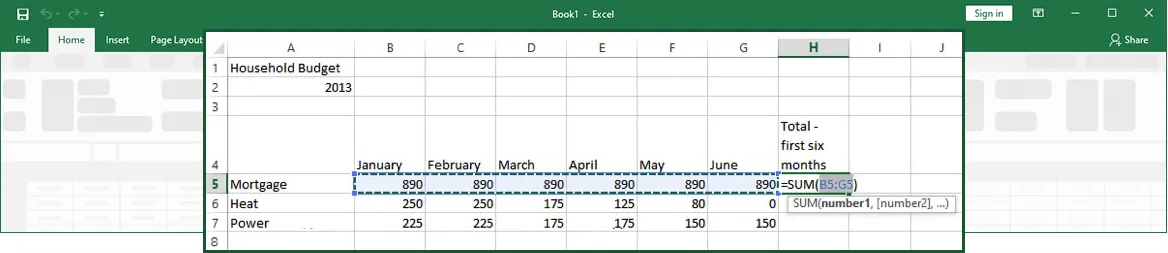1. If the cell references are not accurate, you can drag the highlighted area to include additional cells or remove cells you do not want used in the function.
2. Press ENTER to complete the function.

Excel performs the calculation and moves to the next row. In the following illustration, the cell with the function is active, so that you can see the function syntax in the Formula Bar and the result in the cell.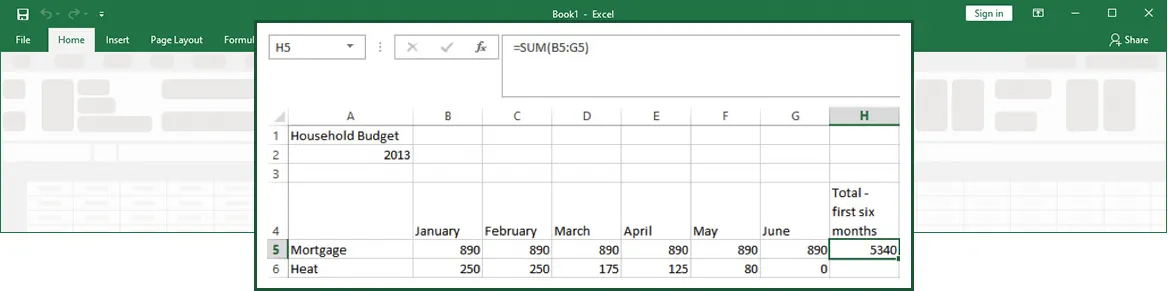#### Using AutoComplete

To use the AutoComplete feature, use the following procedure.

1. Begin typing the SUM function. As soon as you type the Equals sign and the letter S, Excel displays a possible list of matching functions.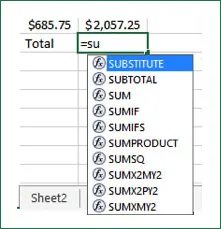1. To select the SUM Function from the list, double-click on the SUM function.
2. Excel enters the function, but you must still enter the arguments. You can simply click on multiple cells, or click and drag to select a cell range. You can also type in the cell references.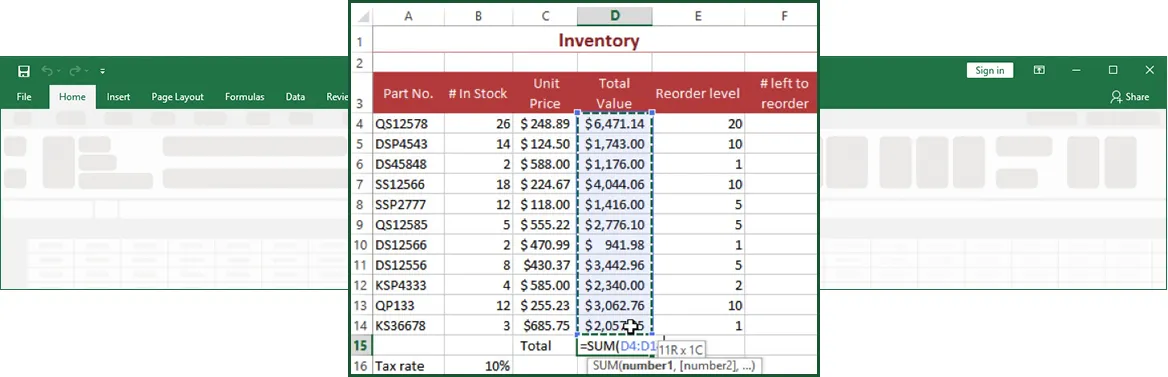1. Enter the final parenthesis mark to end the function.
2. Press ENTER to enter the function in the cell.

#### Using Other Basic Excel Functions

Review how to use the AVERAGE function as an example of another function, use the following procedure.

1. Add a new label in column I: Average.
2. Select the cell in the Average column for the first category.
3. Select the arrow next to the SUM function on the Home tab of the Ribbon to see the list of other common functions.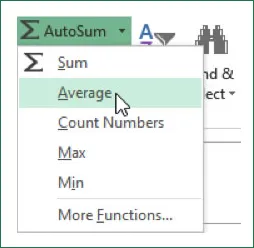1. Select Average. Excel enters the function with the most likely cell references.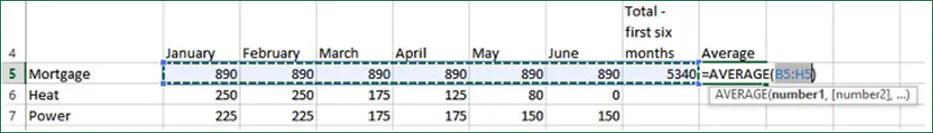1. Replace the cell references so that cell H5 is not included in the average.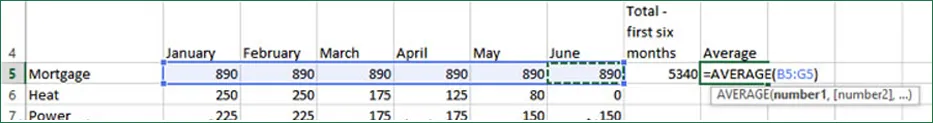1. Press ENTER to complete the function.

#### Group Excel training

Through our network of local trainers we deliver onsite group Microsoft Excel training right across the country. View our Excel training testimonials or obtain a quote for a customized Excel class.